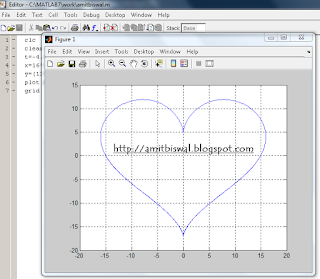How to make a Heart Curve using Matlab Programming?

Well today I am not in any mood to write completely technical stuff, so I thought of doing something different. Some of you may know that valentine day is approaching, so I thought of creating a heart using Matlab programming tools.

To draw a heart shaped curve in Matlab, Just follow the steps given below:

• Start the Matlab software in your computer or laptop.
• Now open a new m-file using File > New > M-File.
• Copy the following set of programs written below and simply paste it in your editor
• ``` ```
`clc`
`clear all`
`t=-10:0.01:10;`
`x=16*(power(sin(t),3));`
`y=(13*cos(t))-(5*cos(2*t))-(2*cos(3*t))-(cos(4*t));`
`plot(x,y)`
• Now run the program (press F5 or you can do it using Debug > Run).
• Your heart shaped curve will be shown in the graph window of Matlab.Heart shaped curve using Matlab Program

Explanation of the program:
Well in this program, we have done nothing but plotted graph between 2 curves that are x and y here, each of which is a combination of few trigonometric equations. You can play around by changing the values of the constants used in these equations and find various shapes of the heart with different shapes of curve.

For a perfect heart figure, the above given constant are optimum to be used in the above used equations (thanks to Weisstein, Eric.W from Wolfram Research Inc)

Well do comment below to let me know if you have any queries regarding this tutorial. Do not forget to share if you liked this tutorial.

Wishing you a Happy Valentine Day in advance!

1.?

2.Great tip! Thanks a lot!

3.Oh Matlab.. make me dizzy.hehe good info anyway!

4.Great. this programming tips is very nice. Thanks

gorilla safari

5.Anonymous3/12/2012

This comment has been removed by a blog administrator.

6.Just a question, how did you remove the nofollow?

7.@kashaya: Just disable the threaded commenting system

8.This is really sweet for Engineer couples. How did you find out about that equation? Or did you create that one yourself? Anyways, your post is good and perfect for the holiday :)

1.I have mentioned the reference for the equation...Thanks

If you liked this blog then Join me at: